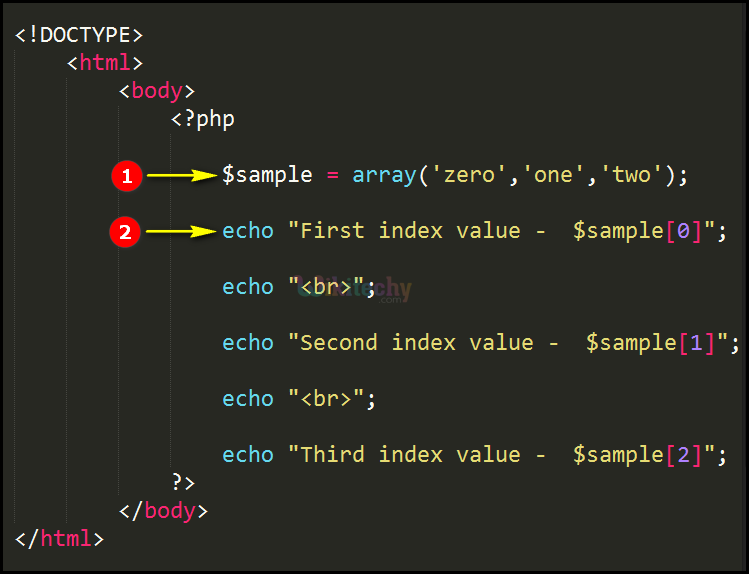# php tutorial - PHP Indexed Arrays - php programming - learn php - php code - php script

• In php the indexed arrays starts with the numeric values that is ‘keys’, which are indexed in which the index value starts with zero (0).
• The index values will be assigned automatically or you can assign manually also.

## php program Code Explanation :1. In this statement we define the indexed array for the variable “\$sample” by using array function and the value are separated by using commas (,).
2. In this echo statement we print the content “first index value and “\$sample ” variable value. In the same way remaining two echo statements will be printed.

## php coding Sample Output :1. For the array “sample” the index value “0” prints the echo statement "First index value -Zero”.
2. for the array “sample” the index value “1” prints the echo statement "Second index value -One”.
3. For the array “sample” the index value “2” prints the echo statement "Third index value -two”.